# LeetCode 70爬楼梯&71简化路径&72编辑距离(dp)

LeetCode 67二进制求和&68文本左右对齐&69x的平方根

## LeetCode 70爬楼梯public int climbStairs(int n) {
if(n<3)return n;
int dp[]=new int[n+1];
dp=1;
dp=2;
for(int i=3;i<n+1;i++)
{
dp[i]=dp[i-1]+dp[i-2];
}
return dp[n];
}


## LeetCode 71 简化路径

输入："/home/"



输入："/../"



输入："/home//foo/"



输入："/a/./b/../../c/"



输入："/a/../../b/../c//.//"



输入："/a//bc/d//././/.."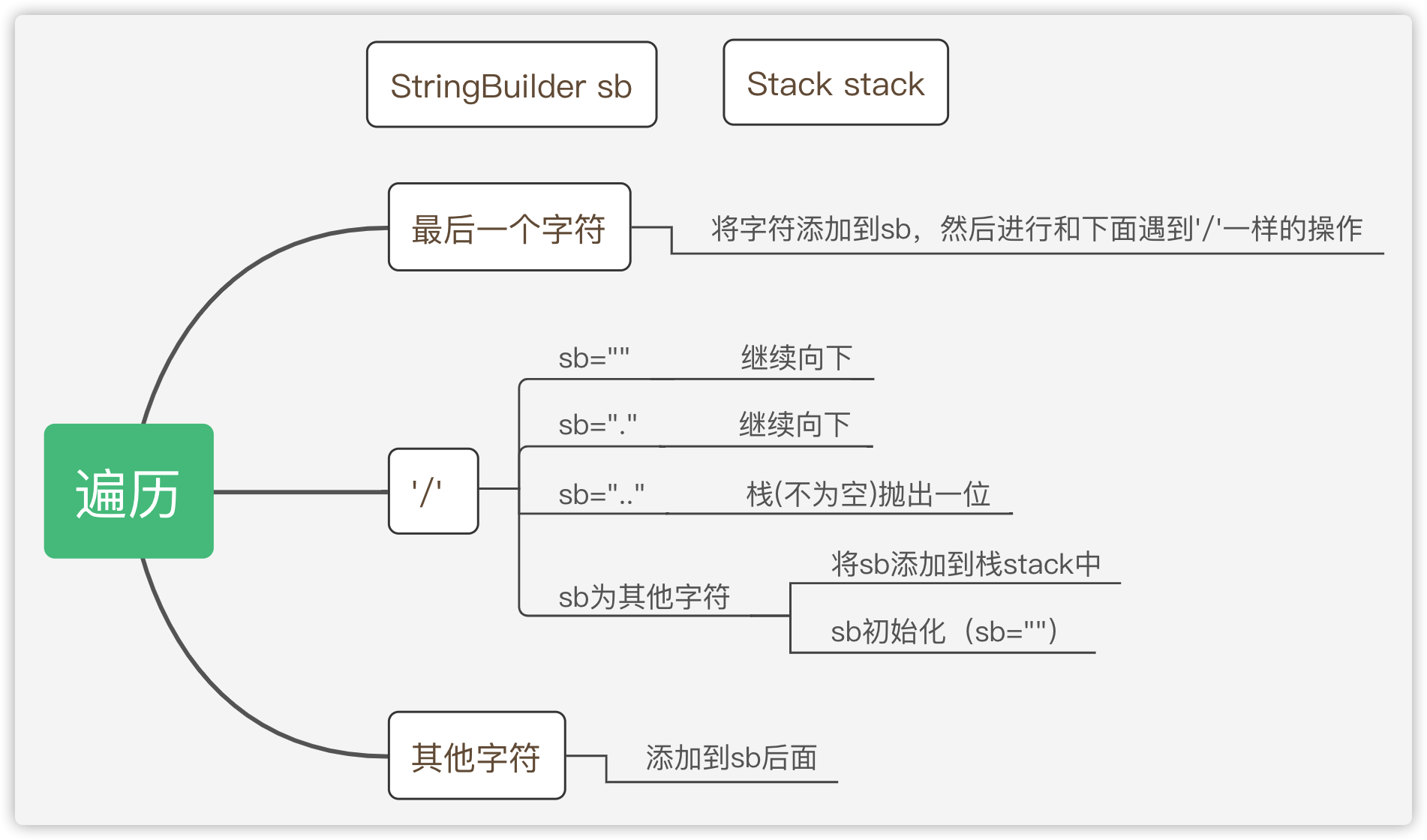public String simplifyPath(String path) {
Stack<String>stack=new Stack<String>();
char ch[]=path.toCharArray();
StringBuilder sBuilder=new StringBuilder();

for(int i=0;i<ch.length;i++)
{

if(ch[i]=='/'||i==ch.length-1)
{
if(i==ch.length-1&&ch[i]!='/')
{
sBuilder.append(ch[i]);
}
if(sBuilder.length()==0||sBuilder.toString().equals("."))
{}
else if (sBuilder.toString().equals("..")) {
if(!stack.isEmpty())
stack.pop();
}
else if(sBuilder.length()>0){
stack.push(sBuilder.toString());
}
sBuilder=new StringBuilder();
}
else
{
sBuilder.append(ch[i]);
}
}

sBuilder=new StringBuilder("");
for(String s:stack)
{
sBuilder.append('/');
sBuilder.append(s);
}
if(stack.isEmpty())
return "/";
return sBuilder.toString();

}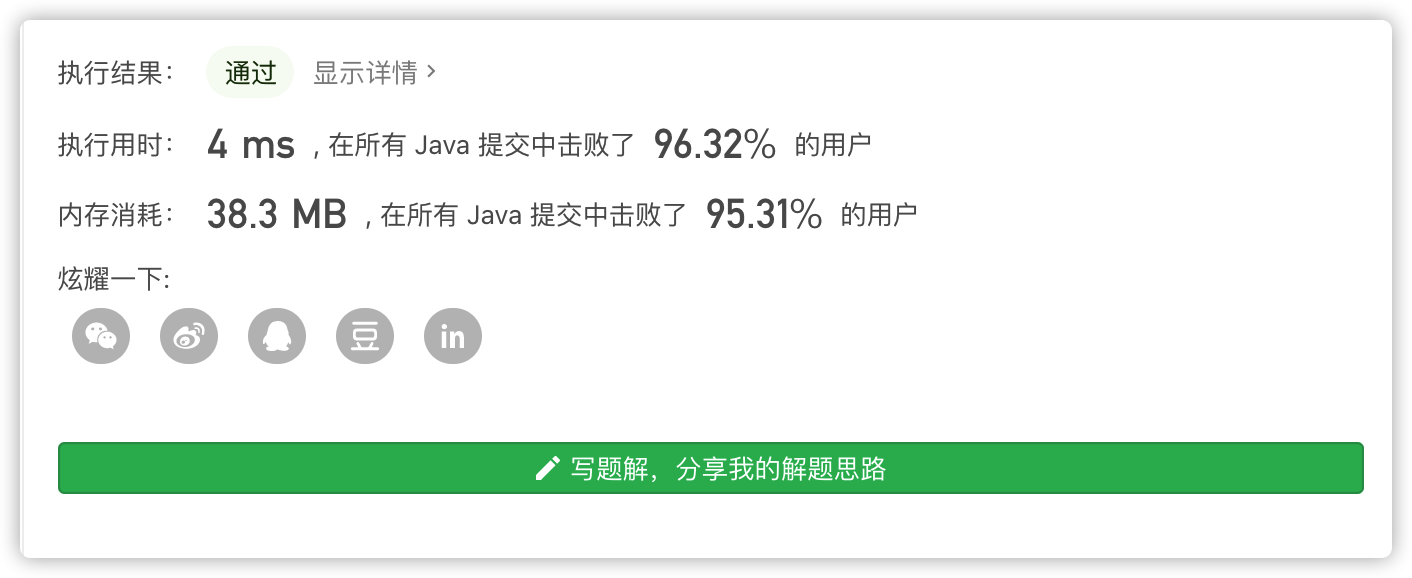## LeetCode 72编辑距离(dp)

输入：word1 = "horse", word2 = "ros"

horse -> rorse (将 'h' 替换为 'r')
rorse -> rose (删除 'r')
rose -> ros (删除 'e')


输入：word1 = "intention", word2 = "execution"

intention -> inention (删除 't')
inention -> enention (将 'i' 替换为 'e')
enention -> exention (将 'n' 替换为 'x')
exention -> exection (将 'n' 替换为 'c')
exection -> execution (插入 'u')


0 <= word1.length, word2.length <= 500
word1 和 word2 由小写英文字母组成


• word1字符串转成word2字符串

• 插入一个字符
• 删除一个字符
• 替换一个字符

• 如果两个字符相等，操作的次数直接向前推。
• 如果不相等，分别递归取最小的(修改，插入，删除)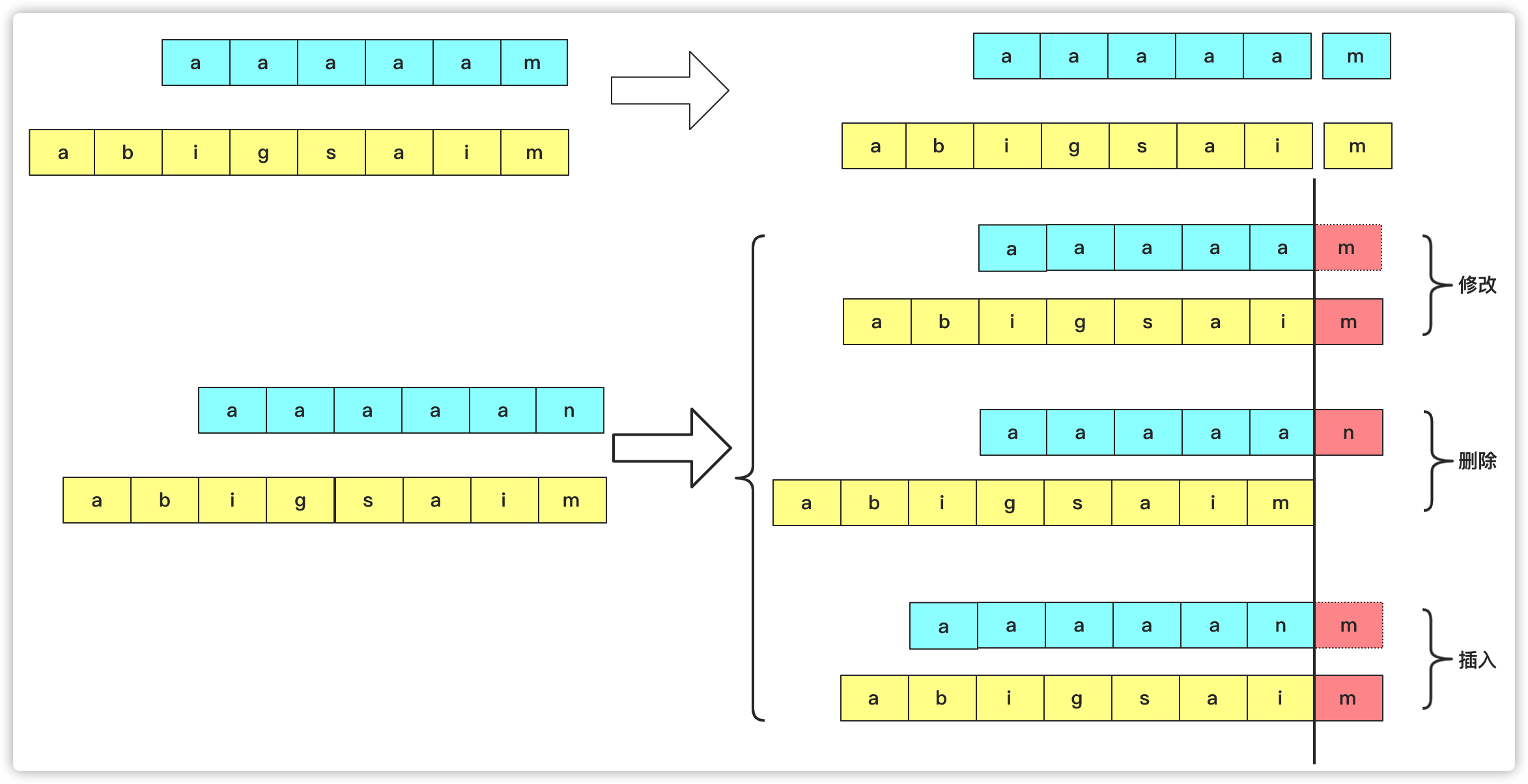• 对于初始化，如果一个串串长度为0，编程另一个串串，那么肯定只有插入和删除这两种操作。并且初始化次数和字符串的长度一致。

• 对于状态转移方程

如果a[i]==b[j]那么说明这个字符相等不需要操作，总次数还是前面a[0-(i-1)]b[0-(j-1)]串操作的次数。

如果a[i]！=b[j]那么就有三种可能取最小的啦并且加一 dp[i][j]=Math.min(dp[i-1][j], Math.min(dp[i][j-1], dp[i-1][j-1]))+1;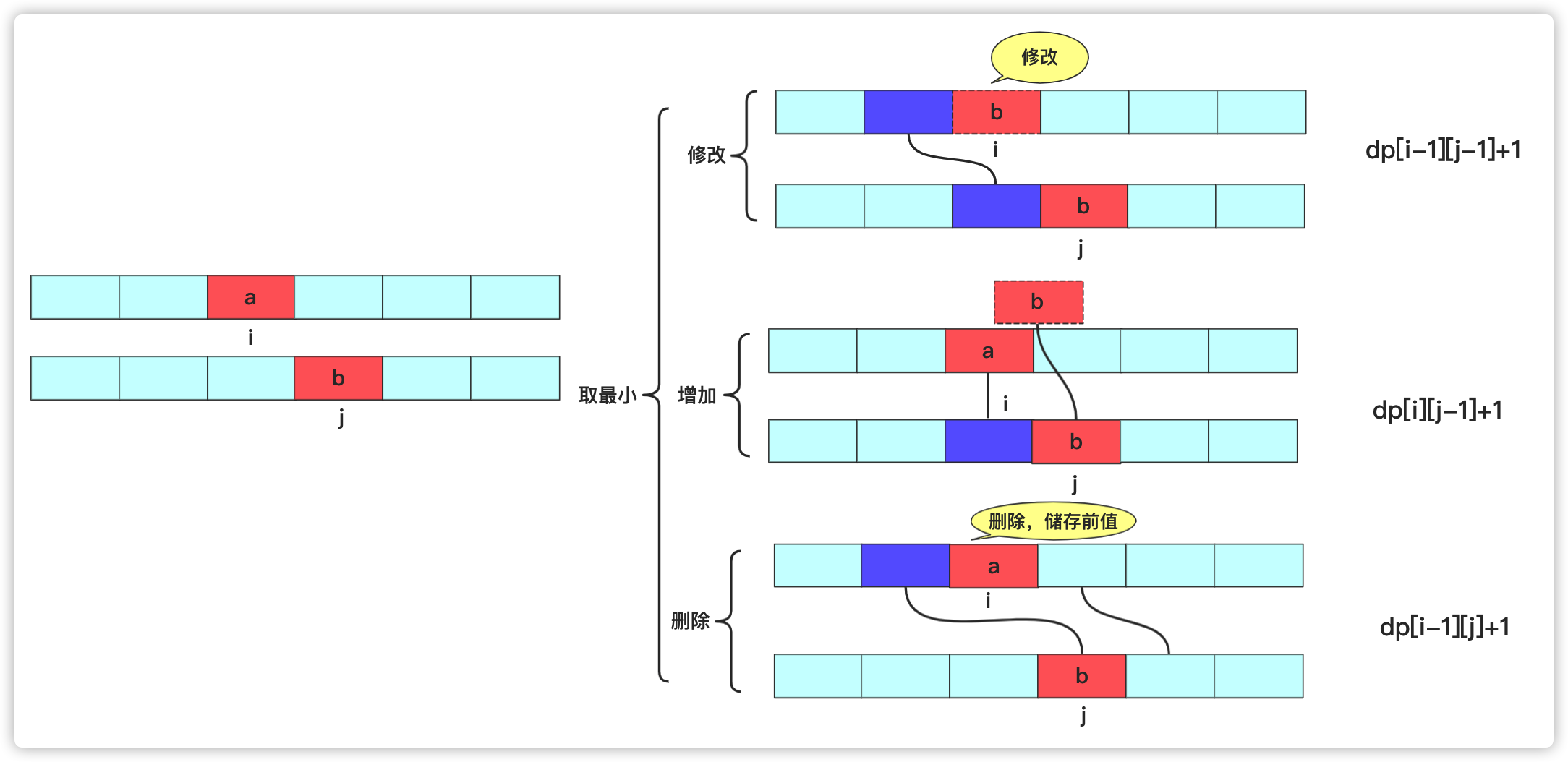public int minDistance(String word1, String word2) {
char ch1[]=word1.toCharArray();
char ch2[]=word2.toCharArray();
if(word1.length()==0)return word2.length();
if(word2.length()==0)return word1.length();
int dp[][]=new int[ch1.length+1][ch2.length+1];
for(int i=1;i<ch1.length+1;i++)
{
dp[i]=i;
}
for(int j=1;j<ch2.length+1;j++)
{
dp[j]=j;
}
for(int i=1;i<ch1.length+1;i++)
{
for(int j=1;j<ch2.length+1;j++)
{
if(ch1[i-1]==ch2[j-1])
{
dp[i][j]=dp[i-1][j-1];
}
else {
dp[i][j]=Math.min(dp[i-1][j], Math.min(dp[i][j-1], dp[i-1][j-1]))+1;
}
}
}
return dp[ch1.length][ch2.length];
}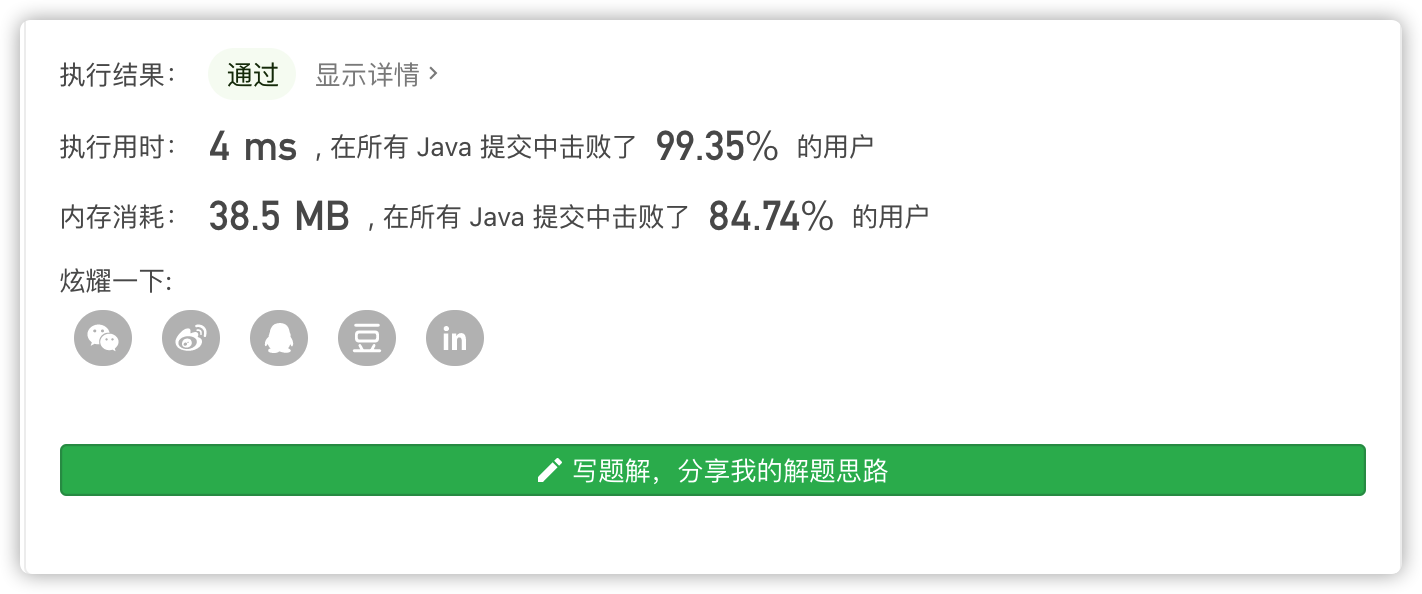## 结语

1. star支持一下， 您的肯定是我在平台创作的源源动力。

2. 微信搜索「bigsai」，关注我的公众号，不仅免费送你电子书，我还会第一时间在公众号分享知识技术。加我还可拉你进力扣打卡群一起打卡LeetCode。Big sai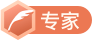CSDN认证博客专家 数据结构与算法 爬虫 Java

10-08197601-211051
03-03581
05-03318
01-27139
12-06583
09-1968
11-291360
12-05584
09-065万+
10-207
07-19161
03-273万+
08-0885
09-283371
10-131万+
02-231万+
03-222万+
02-27268点击重新获取扫码支付余额充值